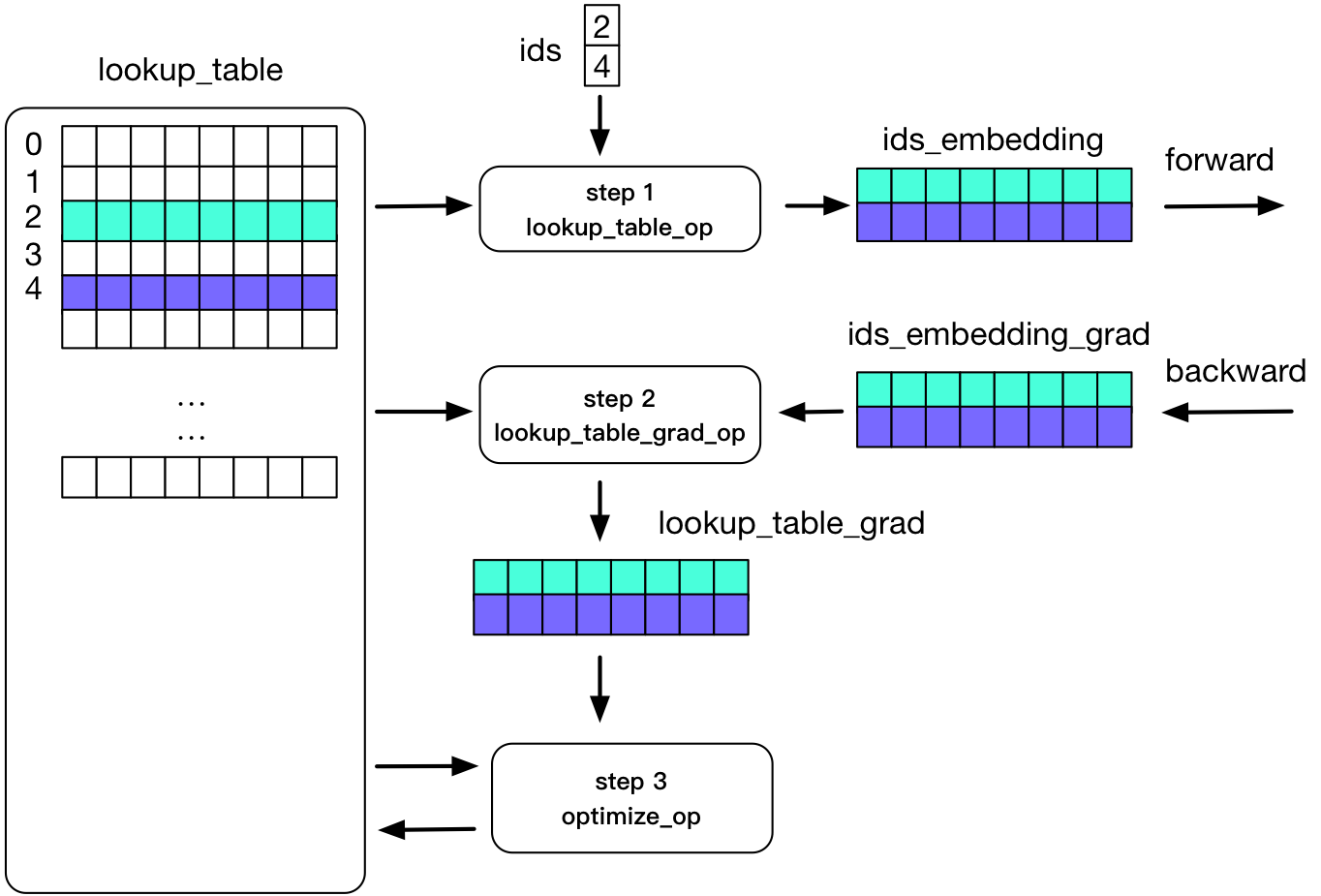# Sparse update¶

Fluid’s api_fluid_layers_embedding layer supports “sparse updates” in both single-node and distributed training, which means gradients are stored in a sparse tensor structure where only rows with non-zero gradients are saved. In distributed training, for larger embedding layers, sparse updates reduce the amount of communication data and speed up training.

In paddle, we use lookup_table to implement embedding. The figure below illustrates the process of embedding in the forward and backward calculations:

As shown in the figure: two rows in a Tensor are not 0. In the process of forward calculation, we use ids to store rows that are not 0, and use the corresponding two rows of data for calculation; the process of backward update is only to update the two lines.## Example¶

API reference api_fluid_layers_embedding . Here is a simple example:

```DICT_SIZE = 10000 * 10
EMBED_SIZE = 64
IS_SPARSE = False
def word_emb(word, dict_size=DICT_SIZE, embed_size=EMBED_SIZE):
embed = fluid.layers.embedding(
input=word,
size=[dict_size, embed_size],
dtype='float32',
param_attr=fluid.ParamAttr(
initializer=fluid.initializer.Normal(scale=1/math.sqrt(dict_size))),
is_sparse=IS_SPARSE,
is_distributed=False)
return embed
```

The parameters:

• `is_sparse` : Whether the gradient is a sparse tensor in the backward calculation. If not set, the gradient is a LodTensor . The default is False.

• `is_distributed` : Whether the current training is in a distributed scenario. Generally, this parameter can only be set in large-scale sparse updates (the 0th dimension of embedding is very large, such as several million or more). For details, please refer to the large-scale sparse API guide 分布式异步训练. The default is False.

• API :
• api_fluid_layers_embedding